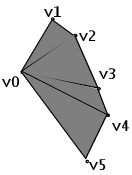Scripting Guide > Three-Dimensional Scenes > Graphics Primitives
Publication date: 08/13/2020

# Graphics Primitives

All scenes in JSL are built with a small number of graphics primitives. These fundamental elements function as the building blocks for complicated scenes.

Every graphics primitive involves specifying vertices. In some cases, the vertices are simply drawn as points. In others, the vertices are connected to form polygons. To draw a primitive, you must specify the type of primitive and the coordinates and properties of the vertices involved. In JSL, this specification is accomplished through the Begin and End statements.

`scene<<Begin(primitive type);`
`...(commands specifying vertices and their properties)...`
`scene<<End();`

To specify the coordinates of the vertices, use the vertex command.

`scene<<Begin(primitive type);`
`scene<<Vertex(x, y, z);`
`...`
`scene<<End();`

The options for primitive type are the following. In these examples, assume that v0, v1, and so on, have been specified between a Begin and End pair, similar to the following.

`scene<<Begin(primitive type);`
`scene<<Vertex(x0,y0,z0) // specify vertex v0`
`scene<<Vertex(x1,y1,z1) // specify vertex v1`
`...`
`scene<<Vertex(xn,yn,zn) // specify vertex vn`
`scene<<End();`
 primitive type=POINTS Draws a point at each of the vertices.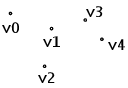primitive type=LINES Draws a series of (unconnected) line segments. Segments are drawn between v0 and v1, between v2 and v3, and so on. If n is odd, the last vertex is ignored.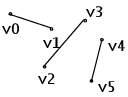primitive type=POLYGON Draws a polygon using the points v0,..., vn as vertices. Three vertices must exist, or nothing is drawn. In addition, the polygon specified must not intersect itself and must be convex. If the vertices do not satisfy these conditions, the results are unpredictable.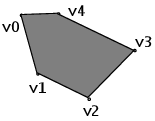primitive type=TRIANGLES Draws a series of (disconnected) triangles using vertices v0, v1, v2, then v3, v4, v5, and so on. If the number of vertices is not an exact multiple of 3, the final one or two vertices are ignored.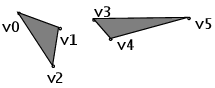primitive type=LINE_STRIP Draws a line segment from v0 to v1, then from v1 to v2, and so on. Therefore, n vertices specify n–1 line segments. Nothing is drawn unless there is more than one vertex. There are no restrictions on the vertices describing a line strip; the lines can intersect arbitrarily.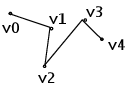primitive type=LINE_LOOP Same as LINE_STRIP, except that a final line segment is drawn from the last vertex to the first, completing a loop.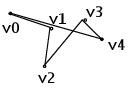primitive type=QUADS Draws a series of quadrilaterals (four-sided polygons) using vertices v0, v1, v2, v3, then v4, v5, v6, v7, and so on. If the number of vertices is not a multiple of 4, the final one, two, or three vertices are ignored.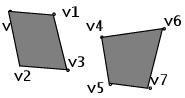primitive type=QUAD_STRIP Draws a series of quadrilaterals (four-sided polygons) beginning with v0, v1, v3, v2, then v2, v3, v5, v4, then v4, v5, v7, v6, and so on. The number of vertices must be at least 4 before anything is drawn, and if odd, the final vertex is ignored.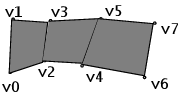primitive type=TRIANGLE_STRIP Draws a series of triangles (three-sided polygons) using vertices v0, v1, v2, then v2, v1, v3 (note the order), then v2, v3, v4, and so on. The ordering is to ensure that the triangles are all drawn with the same orientation so that the strip can correctly form part of a surface. There must be at least three vertices for anything to be drawn.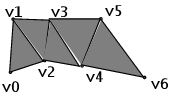primitive type=TRIANGLE_FAN Same as TRIANGLE_STRIP, except that the vertices are v0, v1, v2, then v0, v2, v3, then v0, v3, v4, and so on.